Command Reference : Operator and Function Reference : Statistical Distribution Functions

Statistical Distribution Functions
The following functions provide access to the density or probability functions, cumulative distribution, quantile functions, and random number generators for a number of standard statistical distributions.
There are four functions associated with each distribution. The first character of each function name identifies the type of function:

 Function Type Beginning of Name Cumulative distribution (CDF) @c Density or probability @d Quantile (inverse CDF) @q Random number generator @r
The remainder of the function name identifies the distribution. For example, the functions for the beta distribution are @cbeta, @dbeta, @qbeta and @rbeta.
When used with series arguments, EViews will evaluate the function for each observation in the current sample. As with other functions, NA or invalid inputs will yield NA values. For values outside of the support, the functions will return zero.
Note that the CDFs are assumed to be right-continuous: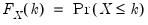. The quantile functions will return the smallest value where the CDF evaluated at the value equals or exceeds the probability of interest: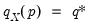, where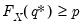. The inequalities are only relevant for discrete distributions.
Many of the cumulative distribution functions take an optional argument at the end of the options list ([,n]). Non-zero values tell EViews to compute the upper tail of the CDF.
The information provided below should be sufficient to identify the meaning of the parameters for each distribution.
Univariate Distributions

 Distribution Functions Density/Probability Function Beta @cbeta(x,a,b[,n]), @dbeta(x,a,b), @qbeta(p,a,b), @rbeta(a,b)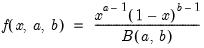for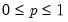and for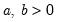, where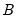is the @beta function. Binomial @cbinom(x,n,p), @dbinom(x,n,p), @qbinom(s,n,p), @rbinom(n,p)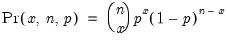if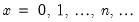, and 0 otherwise, for. Chi-square @cchisq(x,v[,n]), @dchisq(x,v), @qchisq(p,v), @rchisq(v)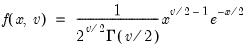where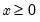, and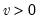. Note that the degrees of freedom parameter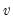need not be an integer. In addition, the @chisq(x,v) function may be used to obtain the p-values directly. Exponential @cexp(x,m[,n]),@dexp(x,m),@qexp(p,m),@rexp(m)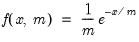for, and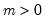. Extreme Value (Type I-minimum) @cextreme(x[,n]), @dextreme(x), @qextreme(p), @cloglog(p), @rextreme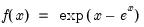for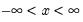. F-distribution @cfdist(x,v1,v2[,n]), @dfdist(x,v1,v2), @qfdist(p,v1,v2), @rfdist(v1,v1)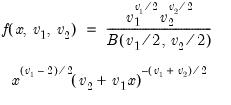where, and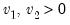. Note that the functions allow for fractional degrees of freedom parameters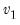and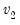. Gamma @cgamma(x,b,r[,n]), @dgamma(x,b,r), @qgamma(p,b,r), @rgamma(b,r)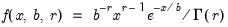where, and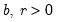. Generalized Error @cged(x,r[,n]),@dged(x,r),@qged(p,r), @rged(r)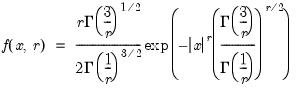where, and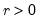. Laplace @claplace(x[,n]), @dlaplace(x), @qlaplace(x), @rlaplace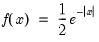for. Logistic @clogistic(x[,n]), @dlogistic(x), @qlogistic(p), @rlogistic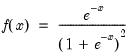for. Log-normal @clognorm(x,m,s[,n]), @dlognorm(x,m,s), @qlognorm(p,m,s), @rlognorm(m,s)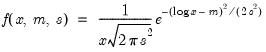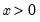,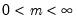, and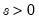. Negative Binomial @cnegbin(x,n,p), @dnegbin(x,n,p), @qnegbin(s,n,p), @rnegbin(n,p)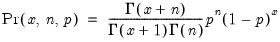if, and 0 otherwise, for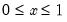. Normal (Gaussian) @cnorm(x[,n]),@dnorm(x), @qnorm(p),@rnorm, nrnd, @logcnorm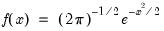for. @logcnorm is a more numerically stable function for the computation of @log(@cnorm(x)) Poisson @cpoisson(x,m), @dpoisson(x,m), @qpoisson(p,m), @rpoisson(m)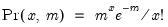if, and 0 otherwise, for. Pareto @cpareto(x,k,a[,n]), @dpareto(x,k,a), @qpareto(p,k,a), @rpareto(k,a)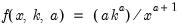for location parameter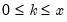and shape parameter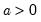. Student's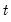‑distribution @ctdist(x,v[,n]), @dtdist(x,v), @qtdist(p,v), @rtdist(v)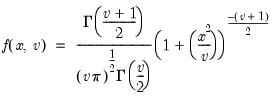for, and. Note that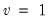, yields the Cauchy distribution. Uniform @cunif(x,a,b),@dunif(x,a,b),@qunif(p,a,b),@runif(a,b), rnd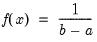for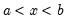and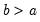. Weibull @cweib(x,m,a[,n]), @dweib(x,m,a), @qweib(p,m,a), @rweib(m,a)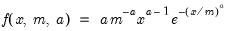where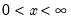, and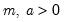.
Bivariate Distributions

 Distribution Functions Density/Probability Function/Additional Info Bivariate Normal Distribution @cbvnorm(x,y,r),@dbvnorm(x,y,r) Compute the bivariate normal cumulative distribution and density functions at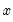and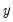for a distribution with means 0, variances 1, and correlation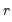.
Multivariate Distributions
For the multivariate normal, Wishart, and inverse Wishart distributions, the basic functions perform a random draw from the distribution or provide the density of the distribution at a point. Distributions are parameterized in part or in full by a scale matrix, which can be supplied in several additional forms as indicated by the function’s suffix.

 Function Suffix Additional Forms for Scale Matrix Input -c Supply the Cholesky decomposition of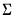. This form is more efficient when performing multiple draws from the same distribution (compute the Cholesky once, but sample many times). -i Supply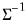. This form is more efficient than explicitly invertingto supply. -ic Supply the Cholesky decomposition of. This form combines the efficiencies of the above forms.
See the table below, which pertains to the multivariate normal and Wishart distributions. For the inverse Wishart distribution, replacewith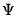Distribution Functions Density/Probability Function/Additional Info Multivariate Normal Distribution @dmvnorm(x, S),@dmvnormc(x, S),@dmvnormi(x, S),@dmvnormic(x, S),@rmvnorm(S[, n]), @rmvnormc(S[, n]),@rmvnormi(S[, n]),@rmvnormic(S[, n])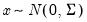, where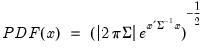and covariance matrixis positive semi-definite.x is a vector, S is a sym object. @dmvnorm* returns a scalar.@rmvnorm* returns a vector or matrix. If the optional argument n is omitted, the function returns a vector containing a single draw from the distribution. If n is provided, n is the number of rows of the returned matrix, with each row representing a draw from the distribution. Wishart Distribution @dwish(X, S, n),@dwishc(X, S, n),@dwishi(X, S, n),@dwishic(X, S, n),@rwish(S, n),@rwishc(S, n),@rwishi(S, n),@rwishic(S, n)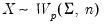, where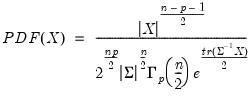,X andare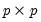positive definite matrices, and the degrees-of-freedom parameter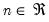and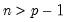.Conceptually, X is the accumulated scatter matrix of n random draws from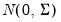, i.e.,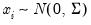,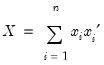,though the mathematical definition has been extended to cover real-valued n.X and S are sym objects, n is a scalar. @rwish* returns a sym object. @dwish* returns a scalar. Inverse Wishart Distribution @riwish(S, n),@riwish(X, S, n),@riwishc(S, n),@riwishc(X, S, n),@riwishi(S, n),@riwishi(X, S, n),@riwishic(S, n),@riwishic(X, S, n),@diwish(X, S, n),@diwishc(X, S, n),@diwishi(X, S, n),@diwishic(X, S, n)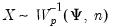, where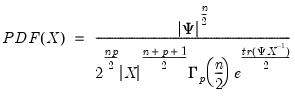,X andarepositive definite matrices, and the degrees-of-freedom parameterand.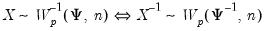, so there are many relations among the Wishart and inverse Wishart functions, e.g., @riwish(S, n) and @inverse(@rwish(@inverse(S), n)) draw from the same distribution.X and S are sym objects, n is a scalar. @riwish* returns a sym object. @diwish* returns a scalar.
 Function Distribution Description @chisq(x,v) Chi-square Returns the probability that a Chi-squared statistic withdegrees of freedom exceeds:@chisq(x,v)=1–@cchisq(x,d) @fdist(x,v1,v2) F-distribution Probability that an F-statistic withnumerator degrees of freedom anddenominator degrees of freedom exceeds: @fdist(x,v1,v2)=1–@cfdist(x,v1,v2) @tdist(x,v) t-distribution Probability that a t-statistic withdegrees of freedom exceedsin absolute value (two-sided p-value): @tdist(x,v)=2*(1–@ctdist(@abs(x),v))